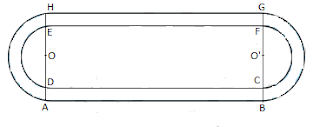Newbie

# Fig. 12.26 depicts a racing track whose left and right ends are semicircular. The distance between the two inner parallel line segments is 60 m and they are each 106 m long. If the track is 10 m wide, find: (i) the distance around the track along its inner edge (ii) the area of the track. Q.8

• 0

In ncert class 10 areas related to circles what is the best solution of exercise 12.3 question no. 8 . Give me the easiest and simplest way to solve this question . Find the best way to solve this question please help me to solve this in a simplest way.Fig. 12.26 depicts a racing track whose left and right ends are semicircular. The distance between the two inner parallel line segments is 60 m and they are each 106 m long. If the track is 10 m wide, find: (i) the distance around the track along its inner edge (ii) the area of the track.

Share

1. Width of the track = 10 m

Distance between two parallel lines = 60 m

Length of parallel tracks = 106 mDE = CF = 60 m

Radius of inner semicircle, r = OD = O’C

= 60/2 m = 30 m

Radius of outer semicircle, R = OA = O’B

= 30+10 m = 40 m

Also, AB = CD = EF = GH = 106 m

Distance around the track along its inner edge = CD+EF+2×(Circumference of inner semicircle)

= 106+106+(2×πr) m = 212+(2×22/7×30) m

= 212+1320/7 m = 2804/7 m

• 0